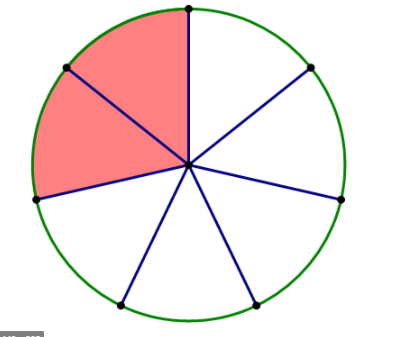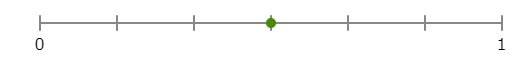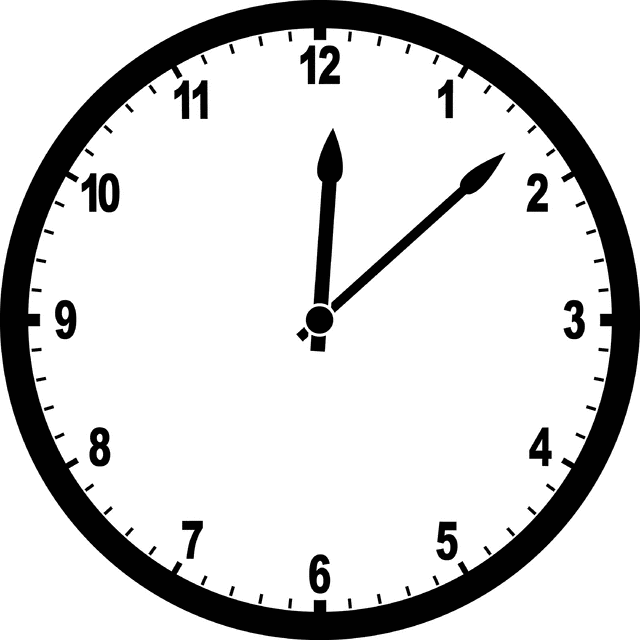Time
100

3 x 6 =

18

100

What fraction is below?2/7

100

Find the Perimeter.40 ft

100

What time is on the clock?10:00

100

Which fraction is greater than 1/2

A. 0/4

B. 1/4

C. 2/4

D. 3/4

D. 3/4

200

42 divided by 6 =

7

200

The circle is partitioned into ________.fourths

200

Find the area.60 cm2

200

What time does the clock show?5:45

200

Are 3/6 and 1/2 equivalent fractions?

Yes

300

9x7=

63

300

4/4 is also equal to what number?

1 whole

300

Sue is buying a rug for her square room. One side of the room has a length of 6 ft. What is the total perimeter of the room?

24 ft.

300

What time is shown on the clock?11:19

300

Are 2/3 and 4/9 equivalent fractions?

No

400

27 divided by 3 =

9

400

What fraction is represented on this number line?3/6

400

Mrs. Banks built a rectangular garden. The width of the garden is 10 meters. Then the length of the garden is 2 times the width. What is the perimeter of the garden in meters?

60 meters

4001:15

400

What number goes in the blank to make the equation true?

_ /8 = 2/4

4

500

12 x 9 =

108

500

_ /5   =  12/20

3

500

The perimeter of a rectangular room is 42 feet. The length is 9 feet. What is the width, in feet, of the floor?

12 feet

50012:08

500

If you have two squares that both show 3/4 shaded in, but one square is bigger than the other, are they equivalent? Why?

No, because the squares have to be the same size. (The whole has to be the same size for both figures.)

Click to zoom